# JP6501406B2 - Measuring device, measuring method, program, and information recording medium - Google Patents

Measuring device, measuring method, program, and information recording medium Download PDF

## Info

Publication number
JP6501406B2
JP6501406B2 JP2016026361A JP2016026361A JP6501406B2 JP 6501406 B2 JP6501406 B2 JP 6501406B2 JP 2016026361 A JP2016026361 A JP 2016026361A JP 2016026361 A JP2016026361 A JP 2016026361A JP 6501406 B2 JP6501406 B2 JP 6501406B2
Authority
JP
Japan
Prior art keywords
acceleration
projectile
acceleration sensor
main
measured
Prior art date
Legal status (The legal status is an assumption and is not a legal conclusion. Google has not performed a legal analysis and makes no representation as to the accuracy of the status listed.)
Active
Application number
JP2016026361A
Other languages
Japanese (ja)
Other versions
JP2017146129A (en
Inventor

Original Assignee

Priority date (The priority date is an assumption and is not a legal conclusion. Google has not performed a legal analysis and makes no representation as to the accuracy of the date listed.)
Filing date
Publication date
Application filed by 国立研究開発法人理化学研究所 filed Critical 国立研究開発法人理化学研究所
Priority to JP2016026361A priority Critical patent/JP6501406B2/en
Publication of JP2017146129A publication Critical patent/JP2017146129A/en
Application granted granted Critical
Publication of JP6501406B2 publication Critical patent/JP6501406B2/en
Application status is Active legal-status Critical
Anticipated expiration legal-status Critical

## Images

•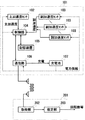•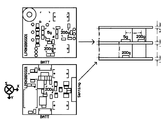••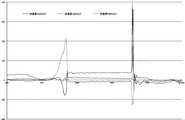•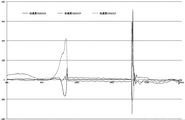•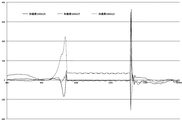•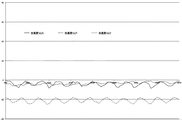•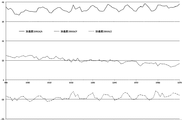•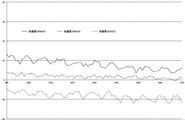•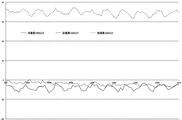•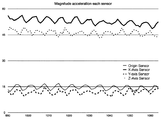•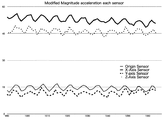•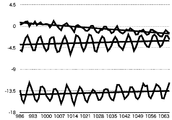••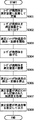## Classifications

• GPHYSICS
• G01MEASURING; TESTING
• G01PMEASURING LINEAR OR ANGULAR SPEED, ACCELERATION, DECELERATION, OR SHOCK; INDICATING PRESENCE, ABSENCE, OR DIRECTION, OF MOVEMENT
• G01P15/00Measuring acceleration; Measuring deceleration; Measuring shock, i.e. sudden change of acceleration
• G01P15/18Measuring acceleration; Measuring deceleration; Measuring shock, i.e. sudden change of acceleration in two or more dimensions
• AHUMAN NECESSITIES
• A63SPORTS; GAMES; AMUSEMENTS
• A63BAPPARATUS FOR PHYSICAL TRAINING, GYMNASTICS, SWIMMING, CLIMBING, OR FENCING; BALL GAMES; TRAINING EQUIPMENT
• A63B43/00Balls with special arrangements
• GPHYSICS
• G01MEASURING; TESTING
• G01PMEASURING LINEAR OR ANGULAR SPEED, ACCELERATION, DECELERATION, OR SHOCK; INDICATING PRESENCE, ABSENCE, OR DIRECTION, OF MOVEMENT
• G01P3/00Measuring linear or angular speed; Measuring differences of linear or angular speeds
• G01P3/42Devices characterised by the use of electric or magnetic means
• G01P3/44Devices characterised by the use of electric or magnetic means for measuring angular speed
• GPHYSICS
• G01MEASURING; TESTING
• G01PMEASURING LINEAR OR ANGULAR SPEED, ACCELERATION, DECELERATION, OR SHOCK; INDICATING PRESENCE, ABSENCE, OR DIRECTION, OF MOVEMENT
• G01P7/00Measuring speed by integrating acceleration
• GPHYSICS
• G07CHECKING-DEVICES
• G07CTIME OR ATTENDANCE REGISTERS; REGISTERING OR INDICATING THE WORKING OF MACHINES; GENERATING RANDOM NUMBERS; VOTING OR LOTTERY APPARATUS; ARRANGEMENTS, SYSTEMS OR APPARATUS FOR CHECKING NOT PROVIDED FOR ELSEWHERE
• G07C1/00Registering, indicating or recording the time of events or elapsed time, e.g. time-recorders for work people
• G07C1/22Registering, indicating or recording the time of events or elapsed time, e.g. time-recorders for work people in connection with sports or games
• AHUMAN NECESSITIES
• A63SPORTS; GAMES; AMUSEMENTS
• A63BAPPARATUS FOR PHYSICAL TRAINING, GYMNASTICS, SWIMMING, CLIMBING, OR FENCING; BALL GAMES; TRAINING EQUIPMENT
• A63B2220/00Measuring of physical parameters relating to sporting activity
• A63B2220/30Speed
• A63B2220/34Angular speed
• A63B2220/35Spin
• AHUMAN NECESSITIES
• A63SPORTS; GAMES; AMUSEMENTS
• A63BAPPARATUS FOR PHYSICAL TRAINING, GYMNASTICS, SWIMMING, CLIMBING, OR FENCING; BALL GAMES; TRAINING EQUIPMENT
• A63B2220/00Measuring of physical parameters relating to sporting activity
• A63B2220/40Acceleration
• A63B2220/44Angular acceleration
• AHUMAN NECESSITIES
• A63SPORTS; GAMES; AMUSEMENTS
• A63BAPPARATUS FOR PHYSICAL TRAINING, GYMNASTICS, SWIMMING, CLIMBING, OR FENCING; BALL GAMES; TRAINING EQUIPMENT
• A63B2225/00Other characteristics of sports equipment
• A63B2225/50Wireless data transmission, e.g. by radio transmitters or telemetry
• A63B2225/52Wireless data transmission, e.g. by radio transmitters or telemetry modulated by measured values

## Description

The present invention relates to a measuring device for measuring the number of revolutions per unit time of a projectile, a measuring method, a program for realizing these by a computer, and a non-temporary computer readable information record in which the program is recorded. It relates to the medium.

The trajectory of the ball when the pitcher throws the ball is largely governed by the initial velocity vector of the ball, the rotation axis vector, and the number of revolutions per unit time. Therefore, if it is possible to objectively acquire such information, it is possible to evaluate the condition of the pitcher and to give feedback to training.

Here, if a speed gun is used, the magnitude of the initial velocity vector of the ball can be known immediately after the end of throwing.

On the other hand, techniques for measuring the number of revolutions per unit time of a ball have been conventionally proposed. For example, Patent Document 1 proposes a technique for disposing an acceleration sensor near the center of gravity of a ball and measuring acceleration in three axial directions to measure the number of revolutions per unit time of the ball. The relationship between the magnitude of the Magnus force and the magnitude of the air resistance to the speed of the ball is separately measured, and based on the rotational speed of the measurement result and the information of the Magnus force and the air resistance based on the measurement, It is supposed to estimate the time change.

As such, there is a strong demand for objectively and accurately measuring the rotation of various projectiles including balls.

JP 2012-58066 A

However, collecting information such as the air resistance value and the Magnus force of each ball is time-consuming. In addition, in the case where it is desired to measure an arbitrary flying object such as a wedge, a disk, a hammer or the like having an asymmetrical shape, it is difficult to measure such advance information.

Therefore, there is a need for a technique for measuring the movement of a projectile, in particular, the rotation of a projectile in an inertial coordinate system.

In addition to this, when information on magnus force and air resistance value can be collected, there is also a need for a technology to measure the flight of the projectile more accurately, especially in the inertial coordinate system, by further utilizing this information. ing.

Furthermore, there is also a demand for acquiring information on the rotation during flight immediately after the flight object has ended.

The present invention solves the above problems, and a measuring device for measuring the number of revolutions of a projectile per unit time, a measuring method, a program for realizing these by a computer, and a non-temporary recording the program. Computer readable information storage medium.

In the present invention,
The main acceleration sensor is fixed within a predetermined center of gravity error range from the center of gravity of the projectile or the center of gravity,
An auxiliary acceleration sensor is fixed inside the projectile away from the center of gravity,
The measuring device is
Acquiring a main acceleration measured by the main acceleration sensor while the projectile flies and an auxiliary acceleration measured by the auxiliary acceleration sensor while the projectile flies;
From the acquired main acceleration and the acquired auxiliary acceleration, the number of revolutions per unit time of the projectile is estimated with maximum likelihood.

According to the present invention, a measuring device and method for measuring the number of revolutions per unit time of a projectile, a program for realizing these by a computer, and non-temporary computer readable information recording the program A recording medium can be provided.

It is explanatory drawing which shows schematic structure of the measurement unit fixed to the inside of the projectile which concerns on embodiment of this invention, and the measurement apparatus prepared for the exterior of a projectile. It is explanatory drawing which shows the position of the acceleration sensor fixed to a measurement unit. It is a graph which shows the change of each acceleration measured with the main acceleration sensor after starting the throwing operation | movement of a baseball of baseball until it catches. It is a graph which shows the change of each acceleration measured with the subacceleration sensor (X-axis direction) after starting throwing operation of a baseball of baseball until it catches. It is a graph which shows the change of each acceleration measured with the subacceleration sensor (Y-axis direction) after starting throwing operation of a baseball of baseball until it catches. It is a graph which shows the change of each acceleration measured with the subacceleration sensor (Z-axis direction) after starting throwing operation of a baseball of baseball until it catches. It is a graph which shows the change of each acceleration measured by the main acceleration sensor while the baseball of a ball is flying. It is a graph which shows the change of each acceleration measured by the subacceleration sensor (X-axis) while the ball of baseball is flying. It is a graph which shows the change of each acceleration measured with the subacceleration sensor (Y-axis) while the ball of baseball is flying. It is a graph which shows the change of each acceleration measured by the subacceleration sensor (Z-axis) while the ball of baseball is flying. It is a graph which shows the time change of the size of the acceleration measured by each acceleration sensor. It is the graph which matched the phase of the time change of the magnitude | size of the acceleration measured by each acceleration sensor. It is a graph which shows the increase / decrease trend of the time change of each component of the acceleration measured by the main acceleration sensor, and a trend straight line. It is a graph which shows the increase / decrease trend of a time change of each component of the acceleration measured by the main acceleration sensor, and a trend curve. It is a flowchart which shows the execution procedure of the measuring method which concerns on this embodiment.

Hereinafter, embodiments of the present invention will be described. In addition, this embodiment is for description, and does not limit the scope of the present invention. Therefore, one skilled in the art can adopt an embodiment in which each element or all elements of the present embodiment are replaced by equivalent ones. In addition, the elements described in each embodiment can be appropriately omitted depending on the application. Thus, any embodiment constructed according to the principles of the present invention is within the scope of the present invention.

(Measurement unit)
In the present embodiment, the measurement unit is fixed to the center of gravity of the projectile. FIG. 1 is an explanatory view showing a schematic configuration of a measurement unit fixed to the inside of a projectile according to an embodiment of the present invention and a measurement device prepared outside the projectile. This will be described below with reference to this figure.

In the measurement unit 101, two types of three-axis acceleration sensors, a main acceleration sensor 102 and a secondary acceleration sensor 103, are fixed. In the present embodiment, the state of rotation of the projectile is measured by using these acceleration sensors.

In addition, it is possible to measure rotation also using a gyro sensor. However, in general, gyro sensors are large in size and power consumption is also large. On the other hand, as employed in the present embodiment, the acceleration sensor is inexpensive, can be miniaturized, and has low power consumption.

The main acceleration sensor 102 is fixed at or near the center of gravity of the projectile (within a predetermined center-of-gravity error range from the center of gravity). The main acceleration sensor 102 mainly measures the acceleration caused by the movement of the projectile.

The secondary acceleration sensor 103 is fixed to the inside of the projectile at a distance from the center of gravity of the projectile. The secondary acceleration sensor 103 mainly measures the acceleration caused by the rotation of the projectile.

It is desirable to employ at least three high acceleration sensors as the secondary acceleration sensor 103. It is desirable that the three displacement vectors from the main acceleration sensor 102 to the three high acceleration sensors be substantially orthogonal (within a predetermined direction error range) to each other. Also, the lengths of the three displacement vectors can be configured to be approximately equal (within a predetermined length error range).

For example, a baseball ball or golf ball is formed by placing an outer shell after winding or filling an elastic body around a core material. Therefore, the measurement unit 101 may be fixed to the center of the core material. Further, since the cannonball is formed of a uniform metal, the measurement unit 101 may be disposed at the center thereof.

In addition, for hollow balls such as baseball balls, soccer balls, tennis balls, volleyballs, etc., support elastic bodies such as pillars from the outer shell of the ball to the vicinity of the center of gravity, supporting branches, supporting rods, supporting springs, etc. It is sufficient to provide and arrange the measurement unit at the center of the ball.

In addition to this, it is possible to measure the state of rotation by fixing the measurement unit to the center of gravity of a rod, a disk, a hammer, a gun shot, etc. used in sports.

The respective units of the measurement unit 101 are controlled by the control unit 104. The control unit 104 reads the measurement results of the main acceleration and the sub acceleration from the main acceleration sensor 102 and the sub acceleration sensor 103 while the projectile is flying, and records the measurement results in the storage device 105. As the storage device 105, a random access memory (RAM), a solid state disk (SSD), a flash memory, or the like can be employed.

The result recorded in the storage device 105 is transmitted to the outside of the aircraft by near field communication. The control unit 104 controls the communication unit 106 to detect whether near-field wireless communication with the outside is possible, and transmits the measurement result of the acceleration read from the storage device 105 to the outside, if possible.

In addition, charging of the rechargeable battery 107 is performed using an antenna for communication included in the communication unit 106 or a separately prepared circuit or the like.

For example, when the projectile is placed in a constant magnetic field and rotated, power is generated by electromagnetic induction, so that the rechargeable battery 107 may be charged. The rechargeable battery 107 supplies power for operating the main acceleration sensor 102, the secondary acceleration sensor 103, the control unit 104, the storage device 105, and the communication unit 106.

It is also possible to calibrate the measuring unit 101 by performing this rotation at a constant angular velocity. The method of calibration will be described later.

Note that communication and charging may be performed via a connector for wired connection, or the measurement unit 101 may be removed from the flying object to perform communication and charging.

Hereinafter, in order to facilitate understanding, a hardball of baseball will be described as an example of a flying object.

Since the projectile falls freely while the projectile is flying, the acceleration measured by the main acceleration sensor 102 is mainly due to the magnus force and the resistance. Generally, this is often smaller than the acceleration caused by the rotation of the projectile. Therefore, by making the measurable range of the secondary acceleration sensor 103 wider than the measurable range of the main acceleration sensor 102, more accurate measurement becomes possible. It is desirable that the measurable range of the main acceleration sensor 102 and the measurable range of the secondary acceleration sensor 103 be capable of measuring an acceleration according to the moving speed or rotational speed assumed for the projectile. These can be determined by experiment.

For example, in order to investigate how the pitcher rotates the pitched ball of a baseball-style hardball, if the distance between the main acceleration sensor 102 and each of the secondary acceleration sensors (high acceleration sensor) 103 is approximately 7 mm, the main acceleration sensor A low acceleration sensor with a measurement range of about 5 G may be adopted as 102, and a high acceleration sensor with a measurement range of about 200 G may be adopted as the secondary acceleration sensor 103. These numerical values can be suitably changed according to a use or a purpose.

FIG. 2 is an explanatory view showing the position of the acceleration sensor fixed to the measurement unit. This will be described below with reference to this figure.

The main acceleration sensor 102 is arranged at one position "5g" and the sub acceleration sensor 103 is arranged at three positions "200g" on the bottom substrate (lower left of this figure) and middle substrate (upper left of this figure) It is done. The bottom substrate and the middle substrate are separated and fixed as shown in the side view (right in the figure), and in the side view, the main acceleration sensor 102 is separated by an equal distance (7 mm) to the right, in front, and below. , The secondary acceleration sensor 103 is disposed. The measuring unit constructed on the basis of such a substrate is compact and very lightweight, with a weight of about 16 g, and can be incorporated into the core of a baseball rigid ball.

(measuring device)
The measuring device 201 prepared outside the projectile acquires the acceleration measured while the projectile is flying from the measurement unit 101, and estimates the number of rotations per unit time of the projectile, and the like. This measuring device 201 is typically realized by a computer executing a program. The computer is connected to various output devices and input devices to exchange information with these devices.

Programs executed by a computer can be distributed and sold by a server to which the computer is communicably connected, and can be a compact disk read only memory (CD-ROM), a flash memory, or an EEPROM (Electrically Erasable Programmable ROM). And the like, and it is also possible to distribute, sell, etc. the information recording medium after recording on a non-transitory information recording medium.

The program is installed on a non-transitory information recording medium such as a hard disk of a computer, a solid state drive, a flash memory, an EEPROM or the like. Then, the information processing apparatus according to the present embodiment is realized by the computer. Generally, a central processing unit (CPU) of a computer reads out a program from an information recording medium to a random access memory (RAM) under the control of an operating system (OS) of the computer, and then codes included in the program. Interpret and execute. However, in an architecture in which the information recording medium can be mapped in a memory space accessible by the CPU, explicit program loading to the RAM may not be necessary. In addition, various information required in the process of program execution can be temporarily recorded in the RAM (temporary).

Note that the information processing apparatus of the present embodiment can be configured using a dedicated electronic circuit instead of realizing the information processing apparatus of the present embodiment using a general-purpose computer. In this aspect, the program can also be used as a material for generating a wiring diagram or timing chart of an electronic circuit. In such an aspect, an electronic circuit satisfying a specification defined in a program is configured by a field programmable gate array (FPGA) or an application specific integrated circuit (ASIC), and the electronic circuit has a function defined in the program. The device functions as a dedicated device to realize the information processing apparatus of the present embodiment.

In the following, in order to facilitate understanding, the measuring apparatus 201 will be described on the assumption that the computer is realized by executing a program. The measuring apparatus 201 includes an acquiring unit 202 and an estimating unit 203.

The acquisition unit 202 acquires the main acceleration measured by the main acceleration sensor 102 while the projectile is flying, and the subacceleration measured by the subacceleration sensor 103. As described above, it is possible to acquire these pieces of information from the measurement unit 101 disposed in the vehicle by wireless communication.

FIG. 3A is a graph showing a change in each acceleration measured by the main acceleration sensor from the start of a pitching motion of a baseball to the time of catching. FIG. 3B is a graph showing changes in each acceleration measured by the secondary acceleration sensor (in the X-axis direction) from the start of the throwing motion of the baseball to the time of catching. FIG. 3C is a graph showing a change in each acceleration measured by the secondary acceleration sensor (in the Y-axis direction) from the start of the throwing motion of the baseball to the time of catching. FIG. 3D is a graph showing changes in each acceleration measured by the secondary acceleration sensor (in the Z-axis direction) from the start of the throwing motion of the baseball to the time of catching. As shown to these figures, the measurement result of the time change of 12 acceleration components is obtained from four acceleration sensors.

At the moment when the throwing operation is finished (near time axis 980) and the moment the ball is caught (near time axis 1080), part of the acceleration reaches a sharp peak or valley due to the impact. Therefore, it is possible to extract the measurement value of the acceleration in flight from among these.

FIG. 4A is a graph showing changes in each acceleration measured by the main acceleration sensor while the baseball is flying. FIG. 4B is a graph showing changes in each acceleration measured by the secondary acceleration sensor (X axis) while the baseball ball is flying. FIG. 4C is a graph showing a change in each acceleration measured by the secondary acceleration sensor (Y axis) while the baseball is flying. FIG. 4D is a graph showing a change in each acceleration measured by the secondary acceleration sensor (Z axis) while the baseball is flying. From these figures, while the ball is flying (time axis 990-1070), each measured value of acceleration changes up and down in a cycle corresponding to the rotation of the ball, and increases or decreases according to the drag applied to the ball It turns out that it is changing along the trend.

From the main acceleration and the sub acceleration thus obtained, the estimation unit 203 calculates the number of revolutions per unit time (angular velocity) of the projectile, the position of the rotation center in the projectile, and the direction of the rotation axis (in the projectile The rotation axis direction in a fixed coordinate system, the rotation axis direction in an inertial coordinate system, etc. are estimated by maximum likelihood estimation according to a predetermined procedure.

In the following, in order to facilitate understanding of the estimation method, three displacement vectors from the main acceleration sensor 102 to each of the sub acceleration sensors 103 are orthogonal to each other and are described as being separated by an equal distance dL = 7 mm. The direction of each displacement vector is referred to as an X axis, a Y axis, and a Z axis of the measurement unit 101 and a coordinate system (projectile coordinate system) fixed to the projectile.

(Angular velocity / number of revolutions per unit time)
The position vector x in the inertial coordinate system (coordinate system fixed to the ground) of the specific position in the projectile is the position vector x 0 of the rotation center, the radius vector r in the projectile coordinate system from the rotation center, the rotation axis vector l, The rotation matrix M ωt with respect to the angular velocity ω and the time t can be expressed as follows.

If this is differentiated by time, the velocity and acceleration can be obtained as follows. Note that time differentiation is expressed by writing dots on variables.

Here, in the projectile coordinate system, the radius vector r does not change with time, so the result of first-order differentiation of the radius vector r with respect to time becomes a zero vector.

Then, the second derivative of the rotation matrix M ωt can be transformed as follows.

Therefore, the second derivative of the position vector x can be expressed as follows.

Gravitational acceleration g, when the drag acceleration a d, the following relationship is established.

On the other hand, the second derivative of the radius vector r can be expressed as follows by multiplying the inverse of the rotation matrix M ωt from the left.

Note that the inverse matrix of the rotation matrix M ωt is the one rotated back by an angle ωt around the rotation axis vector l. Also, the rotation axis vector l is not affected by the rotation by the rotation matrix M ωt . Therefore, the following relationship is established.

Further, l T r is the inner product of the rotation axis vector l and a radius vector r (l · r).

Now, hereinafter, as subscripts of variables, B is adopted for the main acceleration sensor 102, and X, Y, Z are adopted in each axial direction for the auxiliary acceleration sensor 103. Let the rotational angles of the main acceleration sensor 102 (B) and the sub acceleration sensor 103 (X, Y, Z) about the rotation axis be θ X , θ Y , θ Z. Then, the acceleration (main acceleration) to be measured by the main acceleration sensor 102 (B) and the acceleration (three sub accelerations) to be measured by the sub acceleration sensor 103 (X, Y, Z) are It can be expressed as

If this is time-averaged in one cycle, it can be expressed as follows. The time average is expressed by pulling a bar above the variable.

The time average of the acceleration measured by the main acceleration sensor 102 (B) is subtracted from the time average of the acceleration measured by the secondary acceleration sensor 103 (X, Y, Z).

On the other hand, the displacement vector from the main acceleration sensor 102 (B) to the sub acceleration sensor 103 (X, Y, Z) can be expressed as follows. In the case where the displacement vectors are not orthogonal or the lengths are different, the numerical values of each element of the following formula may be changed accordingly.

When these are displayed as components, they can be expressed as follows. In addition, assuming that the rotation axis vector l does not change with time in the one period, the upper bar is omitted in the component notation l x , l y and l z .

Since the length of the rotation axis vector l is 1, the angular velocity ω can be obtained as follows. The angular velocity ω is a value obtained by multiplying the number of rotations per unit time by 2π (a value obtained by writing 360 degrees of one rotation in radians).

As long sought angular velocity ω is from the 1,4,7 equation [Expression 14], l x, l y , it is possible to calculate the magnitude of l z, l x by reference to the remaining formulas, The signs (plus and minus) of l y and l z can be determined.

In the above calculation method, l x , l y and l z are calculated after finding ω. However, while the number of equations in [Equation 14] is nine, the unknowns are four of ω, l x , l y , and l z , and the number of equations is larger. Therefore, under the constraint that the sum of squares of l x , l y and l z is 1, as the maximum likelihood estimation of the unknowns ω, l x , l y and l z so as to minimize the error Also good.

In addition, although it is ideal that time averaging is performed for one cycle of rotation, it is an object to obtain the cycle (angular velocity ω), and therefore the flying object is not the time average for one cycle of rotation. A time average of all the time during the flight may be taken, or a section for which an angular velocity is desired to be obtained may be appropriately extracted to obtain a time average.

In the above description, the number of secondary acceleration sensors 103 is three, but more secondary acceleration sensors 103 may be prepared. In this case, since the number of constraints obtained for the number of unknowns is excessive, accurate measurement can be performed by performing estimation so as to minimize the error.

(Rotation center in spacecraft coordinate system)
Although the main acceleration sensor 102 is fixed in the vicinity of the center of gravity of the projectile (within a predetermined center-of-gravity error range), the center of rotation of the projectile often deviates from the main acceleration sensor 102. Therefore, the main acceleration and the secondary acceleration measured by the main acceleration sensor 102 and the secondary acceleration sensor 103 are out of phase.

FIG. 5 is a graph showing temporal changes in magnitude of acceleration measured by each acceleration sensor. This will be described below with reference to this figure.

In this figure, an example of the magnitude of the acceleration measured by the main acceleration sensor 102 and the secondary acceleration sensor 103 while the projectile is flying is illustrated, and each measurement result is sequentially from the top in the X-axis direction. Of the secondary acceleration sensor 103, the magnitude of the secondary acceleration of the secondary acceleration sensor 103 in the Z-axis direction, the magnitude of the primary acceleration of the origin by the primary acceleration sensor 102, Y It is the magnitude of the (sub) acceleration of the sub acceleration sensor 103 in the axial direction.

The phase shift of each graph corresponds to the shift angle of the sensor position viewed from the rotational axis direction.

In the example shown in the figure, since the magnitudes of the main acceleration (Origin) and the secondary acceleration in the Y-axis direction are small and the phases are almost the same, it is possible that the rotation axis is almost in the same direction as the Y-axis. Recognize. Therefore, the phase shift of the secondary acceleration in the X-axis direction and the Z-axis direction should be close to 90 degrees.

Since the rotation axis vector l can be calculated by the above method, when the main acceleration sensor 102 and the secondary acceleration sensor 103 are viewed from the rotation axis direction, the angle at which each sensor 102, 103 is arranged around the rotation axis is measured The rotation center can be determined by estimating the rotation center coordinates by maximum likelihood estimation such as the least squares method or the steepest descent method so as to match the phase shift at the specified acceleration as much as possible and minimize the phase error. .

FIG. 6 is a graph in which the phases of the time change of the magnitude of the acceleration measured by each acceleration sensor are aligned. In this figure, the peak positions of the graphs are synchronized because the phase shifts are aligned as much as possible.

(Rotation axis direction in inertial coordinate system)
In the above method, each component of the rotation axis vector in the projectile coordinate system is calculated. Therefore, about what rotation axis the projectile is rotating in the inertial coordinate system, coordinate conversion between the inertial coordinate system and the projectile coordinate system is required.

Here, the main acceleration sensor 102 and the sub-acceleration sensor 103 observe the effects of the centrifugal force due to rotation and the resistance to the projectile (gravity, frictional force to reduce the speed, and magnus force to change the trajectory, etc.). Do.

Here, the centrifugal force should be constant if the angular velocity and the rotation axis do not change. However,
(1) Accelerations to be observed when it is assumed that each sensor is rotating around the rotation center and the rotation axis obtained by the above method
(2) Acceleration actually observed,
, There is a gap. This deviation is due to the fact that the drag is changing with time.

Here, if the angular velocity is constant, the magnitude of the drag is determined according to the velocity of the ball. The direction of drag represents a change in the velocity vector of the projectile in the inertial system.

FIG. 7A is a graph showing an increasing / decreasing trend of the time change of each component of the acceleration measured by the main acceleration sensor and a trend line. FIG. 7B is a graph showing an increasing / decreasing trend of the time change of each component of acceleration measured by the main acceleration sensor and a trend curve. Hereinafter, description will be made with reference to these figures.

In these figures, each component of the acceleration measured by the main acceleration sensor 102 with little contribution of centrifugal acceleration is shown. In these figures, the upper main acceleration component gradually decreases, and the middle and lower main acceleration components decrease once and then gradually increase.

The decreasing and increasing trend can be analyzed by the method of least squares. FIG. 7A illustrates a trend line representing the trend of increase and decrease determined by the method of least squares. FIG. 7B illustrates how the trend is regressed to a quadratic curve by the method of least squares.

The inclination of the trend line and the inclination and change of the trend curve represent the change in the direction of drag in the projectile coordinate system.

Here, the force applied to the projectile while the projectile flies for a short time can be classified into gravity, drag, and magnus.

The drag is a force in the direction opposite to the traveling direction of the projectile, and has the effect of reducing the speed of the projectile, but does not affect the traveling direction of the projectile. Gravity and the Magnus force change the direction of flight of the projectile.

Therefore, the centrifugal acceleration component is removed from [Equation 10] to obtain an external force acceleration.

Furthermore, as shown in FIG. 7B, the external force acceleration is approximated by a quadratic equation using the least squares method for each component of the project object coordinate system in the X, Y, Z directions measured by the main acceleration sensor 102. Do.

Here, a d, t is an external force acceleration vector at time t.

The change Δu in the traveling direction of the projectile in the flying time interval [s, e] of the projectile can be calculated as follows.

Consider a coordinate system to analyze changes in the direction of flight of the projectile. In this coordinate system, coordinate axes are written in lower case letters, such as x-axis, y-axis and z-axis.

First, the z-axis is set to extend in the direction of the vector (-Δu). The direction vector k in the z-axis positive direction can be calculated as follows.
k = (-Δu) / | Δu |

Next, the throwing direction of the projectile is set to the y-axis. The direction vector j in the positive y-axis direction can be calculated as follows.
j = a d, s / | a d, s |

Finally, the direction vector i in the x-axis positive direction is calculated as follows.
i = j × k

Thus, the effects of gravity and magnus on the projectile can be expressed by a coordinate system in which the x-axis, y-axis, and z-axis are defined by the three direction vectors i, j, k. That is, the y-axis represents the traveling direction of the projectile, and the z-axis represents the direction of the combined force of gravity and magnus that changes the traveling direction of the projectile.

(Procedure of measurement)
FIG. 8 is a flowchart showing an execution procedure of the measurement method according to the present embodiment. This will be described below with reference to this figure.

First, the user mounts the projectile on the measurement apparatus 201 by bringing the projectile, in which the measurement unit 101 is fixed inside, close to or connected to the measurement apparatus 201, and charges the measurement unit 101 (step S301). . As described above, when utilizing electromagnetic induction for charging, by rotating the flying object 101 in a fixed magnetic field, power is induced via the antenna for near field communication, and the rechargeable battery 107 Charge the battery. Alternatively, the rechargeable battery 107 may be charged by changing the magnetic field generated on the measuring device 201 side.

Next, the user removes the projectile from the measuring device 201 (step S302). Then, the control unit 104 of the measurement unit 101 detects that communication with the measurement device 201 via the communication unit 106 is not possible, and detects the acceleration by the main acceleration sensor 102 and the secondary acceleration sensor 103 and stores the acceleration. Recording on the device 105 is started (step S303).

After this, the user executes throwing of a flying object, etc. (step S304). Then, the flight vehicle flies in the air. While flying, detection and recording of acceleration is continuing.

Then, the user who has caught or recovered the flying object reattaches the flying object to the measuring device 201 by bringing the flying object close to or connecting to the measuring device 201 (step S305).

Then, the control unit 104 of the measurement unit 101 detects that communication via the communication unit 106 is possible, and stops detection and recording of the acceleration (step S306), and the acquisition unit 202 of the measurement apparatus 201 Start communication. Then, the acquiring unit 202 of the measuring apparatus 201 acquires the acceleration recorded in the storage device 105 from the measuring unit 101 (step S307).

When the acquisition of the acceleration is completed, the estimation unit 203 of the measurement apparatus 201 calculates the angular velocity (number of revolutions per unit time) of the projectile, the time in the projectile coordinate system / inertial coordinate system of the rotation axis vector based on the above method. A change or the like is estimated and output (step S308), and the process ends.

In addition, after acquisition of the acceleration is completed, charging of the rechargeable battery 107 of the measurement unit 101 is started to prepare for the next measurement (see step S301). Further, the charge start timing for the measurement unit 101 may be the time when the flying object is attached to the measurement device 201. With this configuration, charging is automatically performed when acquiring acceleration, so that it is possible to prevent forgetting of charging, and since separate charging is not necessary, measurement is performed after one measurement. You can save time and effort until you do it.

Besides, as described above, charging may not necessarily be charging by electromagnetic induction. For example, a circuit or the like separately prepared other than the communication antenna connected to the communication unit 106 can be used, and communication and charging can be performed via a wired connection connector. Further, the measurement unit 101 may be removed from the flying object to perform communication or charging.

(calibration)
Below, the method to calibrate the measured value of the main acceleration sensor 102 and the subacceleration sensor 103 is demonstrated.

As described above, charging of the measurement unit 101 can be performed by rotating the projectile in a magnetic field. The number of revolutions per unit time of the projectile at this time can be controlled and observed from the outside.

Therefore, the number of rotations per unit time in the externally applied rotation, the main acceleration measured in the rotation measurement unit 101, the time change of the secondary acceleration, the number of rotations per unit time estimated in the measuring device 201, and the inertia By comparing the direction of the rotation axis in the coordinate system, it is possible to calibrate the output values of the main acceleration sensor 102 and the sub acceleration sensor 103 and to correct the error of these positions.

In the above embodiment, one main acceleration sensor 102 is disposed at the origin of the flying object coordinate system, and three sub acceleration sensors 103 are disposed on the X, Y, and Z axes of the flying object coordinate system. The number of sensors 103 may be reduced.

In patent document 1, the measurement result of the 3-axis acceleration sensor arrange | positioned at the gravity center of the projectile is wavelet-transformed, and the rotation speed per unit time is calculated | required.

If the acceleration (time change of three components) measured by the main acceleration sensor 102 is subtracted from the acceleration (time change of three or six components) measured by one or two auxiliary acceleration sensors 103 The acceleration mainly resulting from the rotation can be acquired at a location separated from the rotation center.

The obtained acceleration component is considered to have higher accuracy than the acceleration component measured in Patent Document 1. Therefore, it is possible to obtain the number of rotations per unit time by applying the wavelet transform as in Patent Document 1 as well as by using an analysis method with a small calculation load such as fast Fourier transform, with high accuracy and high accuracy. You can get the number of revolutions per round.

In the above [Equation 15], the X, Y, Z components of the main acceleration measured by the main acceleration sensor 102, and the X component of the subacceleration measured by the subacceleration sensor 103 in the X-axis direction, in order to obtain the angular velocity. The Y component of the secondary acceleration measured by the secondary acceleration sensor 103 in the Y-axis direction and the Z component of the secondary acceleration measured by the secondary acceleration sensor 103 in the Z-axis direction are referred to.

For example, when only one secondary acceleration sensor 103 in the X-axis direction is adopted as the secondary acceleration sensor 103,
(1) A value obtained by subtracting the X component of the main acceleration from the X component of the sub acceleration
(2) A value obtained by subtracting the Y component of the main acceleration from the Y component of the sub acceleration
(3) A value obtained by subtracting the Z component of the main acceleration from the Z component of the sub acceleration
The number of rotations per unit time (reciprocal of the fundamental frequency) can be obtained by subjecting at least one of them to fast Fourier transform to obtain the fundamental frequency. If there are differences in the results by adopting three, the rotation per unit time by selecting one by majority, taking an average, or selecting the one obtained from the component with the largest change in acceleration, etc. You can find the number.

In this aspect, although the direction of the rotation axis can not be sufficiently estimated, the number of rotations per unit time can be accurately determined by a simple configuration with a reduced number of secondary acceleration sensors and by a simpler calculation than the conventional one. .

(Summary)
As described above, the measuring device in the present embodiment
The main acceleration sensor fixed within the gravity center of the projectile or within the predetermined gravity center error range from the gravity center, fixed within the projectile away from the gravity center and the main acceleration measured during the flight of the projectile An acquisition unit for acquiring the acquired secondary acceleration sensor and the secondary acceleration measured during the flight of the projectile;
And an estimation unit configured to perform maximum likelihood estimation on the number of revolutions per unit time of the projectile from the acquired primary acceleration and the acquired secondary acceleration.

Moreover, in the measuring apparatus according to the present embodiment,
The secondary acceleration sensor includes at least three high acceleration sensors,
The three displacement vectors from the main acceleration sensor to the three high acceleration sensors are linearly independent,
The estimation unit may be configured to perform maximum likelihood estimation of an angular velocity vector of the projectile.

Moreover, in the measuring apparatus according to the present embodiment,
The three displacement vectors are orthogonal within a predetermined direction error range,
The magnitudes of the three displacement vectors may be configured to be equal within a predetermined length error range.

Moreover, in the measuring apparatus according to the present embodiment,
The range of the magnitude of the main acceleration that can be measured by the main acceleration sensor includes the range that can be taken by the acceleration related to the movement of the center of gravity during the flight of the projectile,
The range of the magnitude of the secondary acceleration that can be measured by the secondary acceleration sensor includes the range in which the acceleration derived from the rotation of the projectile can be taken while the projectile flies.
The range of magnitude of the secondary acceleration that can be measured by the secondary acceleration sensor can be configured to be wider than the range of the magnitude of the main acceleration that can be measured by the primary acceleration sensor.

Moreover, in the measuring apparatus according to the present embodiment,
The main acceleration and the sub acceleration measured by the main acceleration sensor and the sub acceleration sensor during the flight of the projectile are recorded in a storage device disposed inside the projectile,
The acquisition unit may be configured to acquire the recorded primary acceleration and secondary acceleration from the storage device by near field communication after the flight of the projectile ends.

Moreover, in the measuring apparatus according to the present embodiment,
A control unit disposed inside the projectile and controlling the near field communication and recording and acquisition in the storage device charges the power supplied via the near field communication, and the charging is performed. It can be configured to operate with reduced power.

The measuring method according to the present embodiment is
The main acceleration sensor fixed within a predetermined center-of-gravity error range from the gravity center of the projectile or the gravity center measures the main acceleration during the flight of the projectile,
A secondary acceleration sensor fixed inside the projectile spaced from the center of gravity measures the secondary acceleration while the projectile flies.
The information processing apparatus acquires the measured primary acceleration and the measured secondary acceleration,
The information processing apparatus performs maximum likelihood estimation on the number of revolutions per unit time of the projectile from the acquired primary acceleration and the acquired secondary acceleration.

The program according to the present embodiment is
Computer,
A main acceleration measured by a main acceleration sensor fixed within a predetermined barycentric error range from the barycenter of the projectile or the barycenter while the projectile flies, and a distance from the barycenter during the flight of the projectile An acquisition unit for acquiring an auxiliary acceleration measured by an auxiliary acceleration sensor fixed inside the projectile;
It functions as an estimation unit that performs maximum likelihood estimation of the number of revolutions per unit time of the projectile from the acquired primary acceleration and the acquired secondary acceleration.

The non-temporary computer-readable information recording medium according to the present embodiment records the above program.

According to the present invention, a measuring device and method for measuring the number of revolutions per unit time of a projectile, a program for realizing these by a computer, and non-temporary computer readable information recording the program A recording medium can be provided.

101 measuring unit
102 Main acceleration sensor
103 Secondary acceleration sensor
104 Control unit
105 storage device
106 Communication unit
107 rechargeable battery
201 Measuring device
202 Acquisition unit
203 Estimator

## Claims (9)

1. The main acceleration sensor fixed within the gravity center of the projectile or within the predetermined gravity center error range from the gravity center, fixed within the projectile away from the gravity center and the main acceleration measured during the flight of the projectile An acquisition unit for acquiring the acquired secondary acceleration sensor and the secondary acceleration measured during the flight of the projectile;
A measurement apparatus comprising: an estimation unit that estimates the number of revolutions per unit time of the projectile by the maximum likelihood from the acquired main acceleration and the acquired subacceleration.
2. The secondary acceleration sensor includes at least three high acceleration sensors,
The three displacement vectors from the main acceleration sensor to the three high acceleration sensors are linearly independent,
The measurement apparatus according to claim 1, wherein the estimation unit performs maximum likelihood estimation on an angular velocity vector of the projectile.
3. The three displacement vectors are orthogonal within a predetermined direction error range,
The measurement device according to claim 2, wherein the magnitudes of the three displacement vectors are equal within a predetermined length error range.
4. The range of the magnitude of the main acceleration that can be measured by the main acceleration sensor includes the range that can be taken by the acceleration related to the movement of the center of gravity during the flight of the projectile,
The range of the magnitude of the secondary acceleration that can be measured by the secondary acceleration sensor includes the range in which the acceleration derived from the rotation of the projectile can be taken while the projectile flies.
The range of the magnitude of the secondary acceleration that can be measured by the secondary acceleration sensor is wider than the range of the magnitude of the main acceleration that can be measured by the primary acceleration sensor. Measuring device described in.
5. The main acceleration and the sub acceleration measured by the main acceleration sensor and the sub acceleration sensor during the flight of the projectile are recorded in a storage device disposed inside the projectile,
The said acquisition part acquires the recorded said main acceleration and subacceleration from the said memory | storage device by near field communication after the flight of the said flight body is complete | finished. The measuring device according to 1 or 2.
6. A control unit disposed inside the projectile and controlling the near field communication and recording and acquisition in the storage device charges the power supplied via the near field communication, and the charging is performed. The measuring apparatus according to claim 5, wherein the measuring apparatus operates with the supplied power.
7. The main acceleration sensor fixed within a predetermined center-of-gravity error range from the gravity center of the projectile or the gravity center measures the main acceleration during the flight of the projectile,
A secondary acceleration sensor fixed inside the projectile spaced from the center of gravity measures the secondary acceleration while the projectile flies.
The information processing apparatus acquires the measured primary acceleration and the measured secondary acceleration,
The method according to claim 1, wherein the information processing apparatus performs maximum likelihood estimation on the number of revolutions per unit time of the projectile from the acquired primary acceleration and the acquired secondary acceleration.
8. Computer,
A main acceleration measured by a main acceleration sensor fixed within a predetermined barycentric error range from the barycenter of the projectile or the barycenter while the projectile flies, and a distance from the barycenter during the flight of the projectile An acquisition unit for acquiring an auxiliary acceleration measured by an auxiliary acceleration sensor fixed inside the projectile;
A program characterized in that the program functions as an estimation unit that performs maximum likelihood estimation of the number of revolutions per unit time of the projectile from the acquired primary acceleration and the acquired secondary acceleration.
9. A non-transitory computer readable information recording medium in which the program according to claim 8 is recorded.
JP2016026361A 2016-02-15 2016-02-15 Measuring device, measuring method, program, and information recording medium Active JP6501406B2 (en)

## Priority Applications (1)

Application Number Priority Date Filing Date Title
JP2016026361A JP6501406B2 (en) 2016-02-15 2016-02-15 Measuring device, measuring method, program, and information recording medium

## Applications Claiming Priority (3)

Application Number Priority Date Filing Date Title
JP2016026361A JP6501406B2 (en) 2016-02-15 2016-02-15 Measuring device, measuring method, program, and information recording medium
PCT/JP2017/004361 WO2017141766A1 (en) 2016-02-15 2017-02-07 Measurement device, measurement method, program, and information storage medium
US16/075,433 US20190041424A1 (en) 2016-02-15 2017-02-07 Measurement apparatus, measurement method, program, and information recording medium

## Publications (2)

Publication Number Publication Date
JP2017146129A JP2017146129A (en) 2017-08-24
JP6501406B2 true JP6501406B2 (en) 2019-04-17

# Family

## Family Applications (1)

Application Number Title Priority Date Filing Date
JP2016026361A Active JP6501406B2 (en) 2016-02-15 2016-02-15 Measuring device, measuring method, program, and information recording medium

## Country Status (3)

US (1) US20190041424A1 (en)
JP (1) JP6501406B2 (en)
WO (1) WO2017141766A1 (en)

## Families Citing this family (1)

* Cited by examiner, † Cited by third party
Publication number Priority date Publication date Assignee Title
JP2019084009A (en) * 2017-11-06 2019-06-06 株式会社アクロディア Ball with built-in sensor, and system

## Family Cites Families (7)

* Cited by examiner, † Cited by third party
Publication number Priority date Publication date Assignee Title
JP2007014671A (en) * 2005-07-11 2007-01-25 Toppan Printing Co Ltd Ball and its moving record recognition/display system
JP2008073209A (en) * 2006-09-21 2008-04-03 Seiko Epson Corp Ball for ball game, its behavior evaluation support apparatus, and its behavior evaluation support system
JP5196581B2 (en) * 2009-04-22 2013-05-15 国立大学法人信州大学 Rotation detecting device and method of the spheres
JP2012042299A (en) * 2010-08-18 2012-03-01 Chung-Hua Pan Measuring method for sphere movement route
JP5681936B2 (en) * 2010-09-08 2015-03-11 国立大学法人信州大学 Rotation detecting device and method of the spheres
GB201206827D0 (en) * 2012-04-18 2012-05-30 Jolliffe David V Ball game apparatus
KR101193917B1 (en) * 2012-06-08 2012-10-29 한국항공우주연구원 Measuring method for rotational speed of sphere using accelerometers

## Also Published As

Publication number Publication date
JP2017146129A (en) 2017-08-24
WO2017141766A1 (en) 2017-08-24
US20190041424A1 (en) 2019-02-07

## Similar Documents

Publication Publication Date Title
JP6313260B2 (en) Non-transitory computer readable medium having a configured computer-readable instructions to measure one or more swing parameters during golf swing
US20120295726A1 (en) Golf swing analyzer and analysis methods
US8315822B2 (en) Force measurement system having inertial compensation
KR101565739B1 (en) Movement recognition method, device and movement auxiliary device for ball games
JP5764994B2 (en) The sensor unit and the swing analysis system
CN104524755B (en) Analysis pivot means
US9020197B2 (en) Motion analyzing apparatus
JP5870969B2 (en) Motion analysis apparatus and motion analysis program
US20060276256A1 (en) Sporting equipment provided with a motion detecting arrangement
US20130018494A1 (en) System and method for motion analysis and feedback with ongoing dynamic training orientation determination
US9415290B2 (en) Golf swing analyzing apparatus and method of analyzing golf swing
US20130073248A1 (en) Apparatus and method for employing miniature inertial measurement units for deducing forces and moments on bodies
WO2005118086A1 (en) A swing diagnosis device for use in ball game sports
EP2731091A2 (en) Golf swing analysis device, golf swing analysis system and golf swing analysis method
CN102580298B (en) Motion analysis device and motion analysis method
EP2750773B1 (en) Racket sport inertial sensor motion tracking and analysis
US20140100050A1 (en) Golf swing analyzing apparatus and method of analyzing golf swing
US20050020369A1 (en) Golf club with embedded inertial measurement unit and processing
WO2006004908A2 (en) Real-time measurements for establishing database of sporting apparatus motion and impact parameters
US20150012240A1 (en) Motion analysis device
CN104225890B (en) Motion analysis device
JP2013056074A (en) Swing analysis method
EP2414054A1 (en) System and method for observing the swimming activity of a person
CN103706108B (en) Equipment and golf swing analysis golf swing analysis
US9140717B2 (en) Apparatus and method for identifying and analyzing the free flight dynamics of a body

## Legal Events

Date Code Title Description
A621 Written request for application examination

Free format text: JAPANESE INTERMEDIATE CODE: A621

Effective date: 20190122

A131 Notification of reasons for refusal

Free format text: JAPANESE INTERMEDIATE CODE: A131

Effective date: 20190212

A521 Written amendment

Free format text: JAPANESE INTERMEDIATE CODE: A523

Effective date: 20190218

TRDD Decision of grant or rejection written
A01 Written decision to grant a patent or to grant a registration (utility model)

Free format text: JAPANESE INTERMEDIATE CODE: A01

Effective date: 20190312

A61 First payment of annual fees (during grant procedure)

Free format text: JAPANESE INTERMEDIATE CODE: A61

Effective date: 20190318

R150 Certificate of patent or registration of utility model

Ref document number: 6501406

Country of ref document: JP

Free format text: JAPANESE INTERMEDIATE CODE: R150